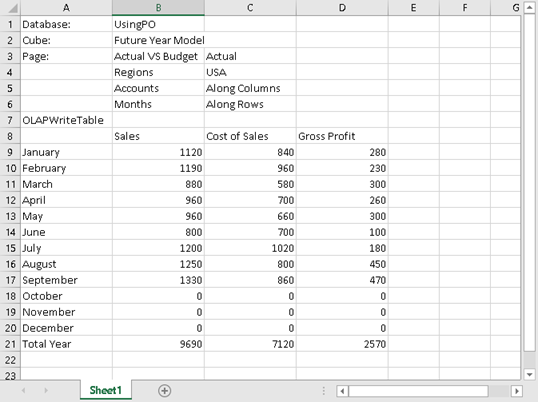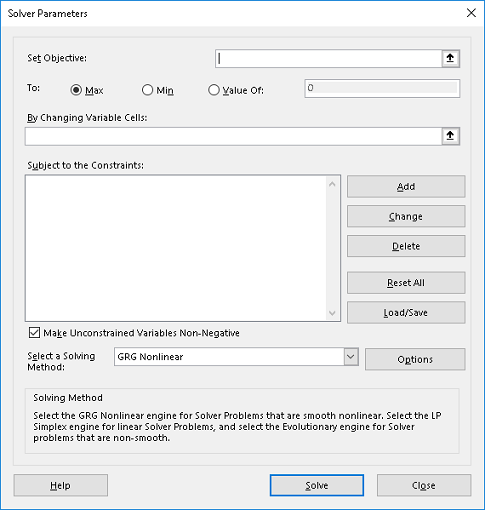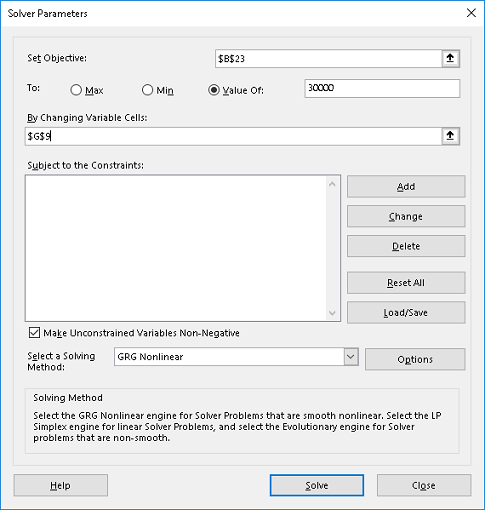## Using Excel Solver in Slice to Excel reportsExcel Solver, or simply 'Solver', is an Excel add-in program which is a tool used for performing what-if analysis and optimizatize or solve problems in models or Excel spreadsheets.

The optimal solution may be achieved by determining tha minimum or maximum values of a certain problem, such as maximum profits and minimum costs, or achieving some target values.

To take advantage of the Excel Solver feature, you need to enable this add-in from within Excel, since this add-in is generally not enabled by default.For instructions on how to Install Excel Solver, click here:

Primary Components of an Optimization Model: Click on the drop-down link to see description.Target CellVariable CellConstraints

Using Solver in an Excel Report: OLAPWriteTable

One example where we might want to use Excel Solver is when working with an Excel report that uses the OLAPWriteTable functionality.

For this particular example, a slice is created out of the Future Year Model cube from the UsingPO database. The objective in the sample demonstration is to compute for the monthly growth rate required, and generate the corresponding target Sales values for each month to achieve the targeted annual Sales value.

The sample demonstration for using the Excel Solver with OLAPWriteTable is as follows:

1.   Open the UsingPO database in PowerOLAP, then create a slice out of the Future Year Model cube with the following Slice configuration:
Page: Actual VS Budget (Actual), Regions (USA)
Columns: Accounts (All)
Months: (all members except the Quarters)

2.   Create an Excel worksheet by going to Slice tab, then clicking on the Worksheet drop down command button and selecting OLAPWriteTable option.Above is a sample Slice to Excel report created from the UsingPO cube using the OLAPWriteTable Excel connection type. To begin, we must first set the OLAPWriteTable function to use or be set into the 'Use Excel Formula' Mode. For this example, a growth rate is specified on a separate cell which will be a basis for computing for the monthly sales values from February till December.

Next, we will define a target annual sales and use the Excel Solver to compute for the required growth rate to be applied each month in order to achieve the desired Total Sales amount for the year.

3.   Double click on the cell containing the OLAPWriteTable function and select 'Use Excel Formulas' as the mode.

4.   On the cell G9, specify the initial growth rate, for this example specify 1.1 as growth rate.

5.   On the cell corresponding to February Sales, create a formula that will multiply the prior month sales (January) to the growth rate (see screenshot below for the formula).

= B9  *  \$G\$9

For this instance, since we will be copying the formula logic to the rest of the months were: Prior Month Sales * Growth rate, make the reference for the cell containing the growth rate an absolute reference.6.   Press F9 or recalculate. Notice that the cell is now marked with a cell/comment indicator.

7.   Copy the formula to cells B11 to B20 (March to December Sales).

8.   Press F9 or recalculate. Notice that all affected cells are marked with cell/comment indicators.

9.   On cell B23 create a formula to sum January to December Sales.

10. Press F9 or recalculate. Notice that the cell is now marked with a cell/comment indicator.Next, use Excel Solver to know what monthly growth rate is needed to achieve a Target annual Sales of \$30,000.

11. From the Excel ribbon, go to Data tab and from the Analysis control group, click on the Solver command button.

Note: If the Solver command cannot be found within the Data tab, it might be because the add-in is not yet installed. To install the Excel Solver add-in program, click on This Link.

The Solver Parameters dialog appears, as show below:12. Begin by setting the value for the target cell, in this case our target cell will be the cell B23, the cell that contains the excel formula which calculates the Total Sales for all months January to December. Next, select the radio button Value Of then type 30000 in the corresponding text box.

13. Next, define our variable cell whose value will be adjusted to satisfy the objective, which in this case is the cell containing the growth rate or cell G9 in the example.14. Click Solve.

15. A message will prompt the screen, with the Keep Solver Solution radio button enabled, click OK.If you go back to the worksheet, notice that the new Growth Rate is now equivalent to 1.3708. Also, notice that since the Sales Values for February to December are formula driven and uses growth rate as one of its factors, these values are also adjusted.16. To commit the calculated values to the database, double click on the OLAPWriteTable function and select the 'Commit formula values to cube' mode. Then to remove the formulas in the worksheet, double click again on the OLAPWriteTable function and select 'Clear formulas and display cube values' mode.